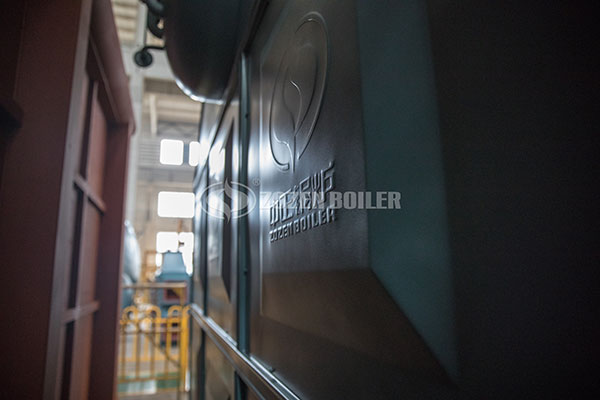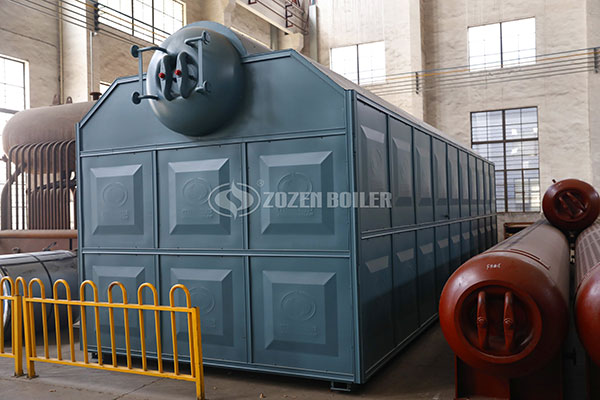# 6 Tons Boiler Cost

March 4, 2020 Tags:

Many users purchase boilers based on the price and quality of the boiler. First of all, the boiler is a special type of equipment, and the quality is in place. When considering the quality and price of the boiler, the later operating cost of the boiler is also a large investment. It is necessary to measure the thermal efficiency and gas consumption of the boiler.

To address the problem of air pollution, the state has introduced some standards to solve the problem. The emission of nitrogen oxides from newly-built boilers in some areas is below 30mg. This requires the use of low-nitrogen boilers, which are relatively expensive. The operating cost of a 6-ton boiler includes: fuel costs, utilities, labor costs, and maintenance costs. Then compare the operating costs of gas boilers and biomass boilers.6-ton gas steam boiler operating cost:

1. Fuel cost: The calculation formula of gas boiler gas consumption is: gas boiler gas consumption (per hour) = gas boiler power * time / fuel calorific value / gas boiler calorific value utilization rate. The power of a 6-ton gas boiler is 4.2MW, the calorific value of the fuel is calculated according to 35.53MJ / Nm3, and the thermal efficiency of the boiler is calculated at 95%. Then, the one-hour consumption of a 6-ton natural gas boiler = 4.2MW * 3600s / 35.53MJ / Nm3 / 95% = 448m3 / h. 3 yuan per ton of natural gas, 1 hour operating cost = 448 * 3 = 1344 yuan.

2. Power consumption: power consumption per hour = (18.5 + 7.5) × 0.65 = 16.9Kw / h, electricity cost is 1 yuan, 16.9 * 1 = 16.9 yuan.

3. Water consumption: 1.1 tons of water per ton of steam, water consumption per hour = 6.6 tons, water fee of 1 yuan, 6.6 * 1 = 6.6 yuan.

4. Workers’ wages: working 8 hours a day, one shift, the salary of a stove worker is 2500 yuan-4000 / month.

5. Daily maintenance costs: 8000 yuan / year for daily maintenance, furnace shutdown and drying, boiler cleaning, etc.6-ton biomass boiler operating cost:

1. Fuel cost: According to a 6-ton boiler, 1080 kg of particles are burned per hour, and the particles are calculated at 1,200 yuan / ton. Fuel cost per hour is 1200×1.08 = 1296 yuan. It costs 10 × 1296 = 12960 yuan for 10 hours per day. Calculate 10368 yuan / day based on 80% of steam volume.

2. Power consumption of motor: drum, induced draft fan 29.5KW, reducer, feeding machine, water pump, conveyor 10KW in total are calculated as 50KW, calculated at 1 yuan per degree. The cost of electricity per hour is 50 × 1 = 50 yuan, 50 × 10 = 500 yuan for 10 hours per day.

3. labor costs: one person per class, divided into 2 classes, RMB 2,000 per person per month.

4. Boiler maintenance and repair costs: Calculated at around 5,000 yuan per year for the first 3 years.

As far as the price of fuel is concerned, biomass fuel is naturally lower than gas; from the perspective of thermal efficiency, biomass boilers are also between coal-fired and gas-fired stoves, which are lower than gas-fired stoves. If calculated comprehensively, the operating cost is also between the two, which is also more cost-effective. If there is a requirement of 30mg of nitrogen oxides, it is recommended to use gas boilers.